telescopeѲptics.net          ▪▪▪▪                                             CONTENTS

# 2.3. Telescope magnification

Telescope magnification is given by a ratio of the image size produced on the retina when looking through a telescope, versus retinal image size with the naked eye. As FIG. 7 shows, image size on the retina in both cases is proportional to the apparent angle of view, giving telescope magnification as MT= ε/α, ε and α being the apparent and true (semi) angle of view, respectively. For sufficiently small ε, the angles relate nearly as their tangents. Replacing the two angles - with their tangents (tanε=h'/fE and tanα=h'/fO) gives telescope magnification as: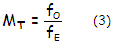with fO, fE being the objective and eyepiece focal length, respectively. For simplicity, both telescope and eyepiece focal length will be considered numerically positive. Also, since most telescope objectives form reversed objefct image, which is not changed by the eyepiece, their magnification is, by definition, numerically negative; for simplicity, it will be given as numerically positive here, since it is not used for other (broader) calculations.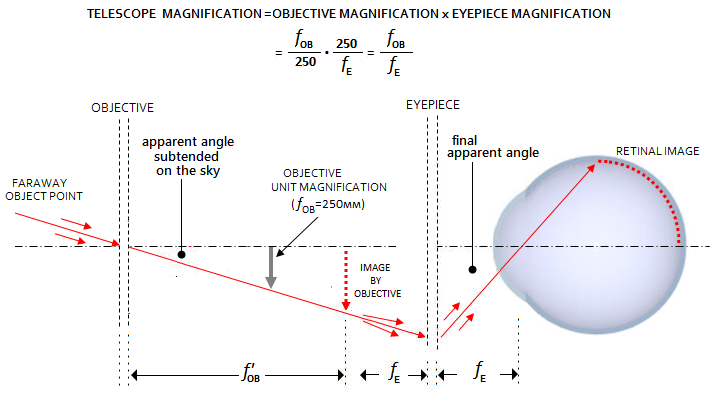Since this relation assumes small angle of view, it is strictly accurate only for small angular objects, not larger than about 10 degrees in the eyepiece. With the tangent increasing faster than the angle, large angular objects in the eyepiece will have lower actual magnification than indicated by this formula. For instance, a 1 arc minute object magnified to 1° apparent size will have actual magnification of 60, exactly as the formula indicates. But a 30 arc minute object magnified to 30° apparent size will also have actual magnification of 60, while the formula indicates 61.4.

Telescope magnification can be split into two components: (1) magnification of the objective and
(2) magnification of the eyepiece. Magnification of the image formed by the objective is either relative to the object imaged (absolute, or optical magnification), or relative to its apparent size in the naked eye (apparent magnification). The former is expressed with a simple formula: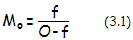with f being the telescope focal length, and O the object distance (FIG. 7). Obviously, it is very small for astronomical objects, due to their enormous distances. Apparent magnification of the objective is given by the ratio of the viewing angle of its object-image from the least distance of distinct vision (250mm average) to the viewing angle of the object observed directly. Since these angles are sufficiently small, they can be replaced with their tangents, giving the apparent objective magnification as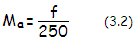for the focal length f in mm.

In terms of image-to-objective separation I (also assumed numerically positive) the apparent telescope magnification is Ma=(I-f)/f, which determines the object distance in terms of I and f as O=If/(I-f). Due to enormous distances of astronomical objects - thus with I only negligibly greater than f - their absolute magnification in a telescope approaches zero.

The eyepiece acts as a magnifying glass, effectively allowing the eye to observe the object-mage formed by the objective from the distance of eyepiece's focal length (fE in FIG. 7). It makes the image apparently larger by a factor ME= ε/β, with ε and β being the apparent viewing angle in the eyepiece and in the naked eye (the latter also called "true" angle, or field of view), respectively (FIG. 6-7). Again, for small to moderate viewing angle ε, we can replace angles with their tangents (tanβ=h'/V~h'/250 for h' in mm), giving the eyepiece magnification factor as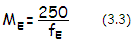for the eyepiece f.l. fE in mm. Thus, apparent telescope magnification is a product of the two magnifications - the initial apparent magnification by the objective, and the final one by the eyepiece - resulting in Mt=MaMe~f/fE. Of course, the eyepiece magnifies by enlarging the apparent angle of rays converging toward the eye, but it produces collimated beams and, thus, no actual image. It is the eye that focuses these collimated beams into point images.

As noted, for large viewing angles in the eyepiece, use of the tangent results in higher than actual magnification figure. For instance, a 5mm high object-image on the optical axis is viewed through a 50mm f.l. eyepiece at an angle α given by tanα=5/50, giving α=5.7 degrees apparent viewing angle. And that same object-image observed through a 10mm f.l. eyepiece has the apparent viewing angle α'=26.6 degrees. While the tangent (i.e. eyepiece f.l.) based magnification factor is 5, actual magnification factor for the 10mm vs. 50mm eyepiece is 26.6/5.7=4.7.

While there is no single optimum magnification for all types of astronomical objects and individuals, there is a range of so called useful magnification. On the low side of this range, the limit is set by the size of eye pupil. It is not to be smaller than the "exit pupil" of a telescope - an image of the entrance pupil (objective) formed by the eyepiece. Exit pupil appears as a bright circle of light floating in front the eyepiece eye lens (the eyepiece lens facing the eye). In order to capture all the light entering telescope, the eye lens has to be placed at the location of exit pupil and, of course, in order to avoid light loss, eye pupil has to be at least as wide as the exit pupil. Since the exit pupil diameter is given by P=D/Mt=fE/F, the lowest magnification that still preserves light-gathering power is Mt=D/Pmax. For the average eye pupil maximum of 6mm, it comes to D/6 for D in mm.

Limit to magnification increase is set primarily by image imperfections, but also by dimming, loss of field, vibrations and eye physiology. As we have seen, even perfect optics will not produce perfect images, due to the effect of diffraction. Point-object image is smeared into a pattern of finite size. Magnified enough (to about 4 to 5 arc minutes) it becomes visible to the eye, and there is no benefit in resolution from further magnification increase. Taking the standard resolution limit for a pair of near equally bright point sources of 4.5/D (D in inches) arc seconds (1/13D in arc minutes), needed magnification to 5 arc minutes apparent center separation for a pair of relatively bright point-object images at the resolution limit is given as ~67D, or 67x per inch of aperture (~2.7D for D in mm).

The often quoted 50x per inch of aperture limit to useful magnification dates back to the 1940s, when Allyn Thompson used a mixture of theoretical diffraction resolution limit for point-like sources, anecdotal accounts of the limit to naked-eye stellar resolution (ε Lyrae), and the results of group's own experiments with naked-eye resolution limits for pairs of 0.0003-inch illuminated pinholes, to come up with four arc minutes as the approximate average resolving limit for point-like objects (Making your own telescope, p173-174). Combining it with the Dawes' limit formula for the minimum resolvable stellar separation in arc seconds α=4.56/D for the aperture D in inches, for the corresponding magnification M needed to enlarge this limit to four arc minutes (i.e. 240 arc seconds) apparent size, produced M=240/α=52.6D.

In line with this, higher magnifications than ~50x per inch of aperture would not produce additional benefit.

However, Thompson's consideration missed to recognize that telescopic and naked-eye point-source resolution are distinctly different. The former is limited by diffraction, and the latter by aberrations. At 4mm pupil diameter, which was the estimated pupil size level in their experiments with pinhole resolution (the naked-eye pupil size while resolving ε Lyrae is at least as large), eye not corrected with ophthalmic lenses averages about 1 wave RMS of (mainly) combined defocus and astigmatism. The resulting diffraction blur is roughly a dozen times larger than the Airy disc and, more importantly, its angular size is about 8 arc minutes, decidedly larger than the maximum angular size still perceived by the eye as point-object.

Unlike the naked-eye observer, telescope user has the benefit of eye defocus error corrected by refocusing the eyepiece. This reduces the RMS error by a factor of four, or so, with the diffraction blur shrinking nearly as much, to about 3 arc minutes (FIG. 236A). Hence the average telescope user would need only about 1/3 of the magnification calculated by Thompson - about 17x per inch of aperture - for the theoretical resolution of two point objects at the Dawes' limit. The corresponding eyepiece exit pupil - and the effective eye pupil - is about 1.5mm in diameter, at which the average eye is better than diffraction-limited, and telescopic eye - due to corrected eye defocus error - decidedly diffraction limited. However, since the FWHM angular diameter at this magnification level is still only 1.26 arc minutes, or about 2.5 arc minutes for two touching FWHMs combined (lengthwise), the image is still too small for the eye to clearly discern its shape. For that, the combined image needs to be further enlarged to about 5 arc minutes (~34x per inch magnification). Increase in magnification up to twice as much (70x per inch, with each FWHM enlarged to about 5 arc minutes) still results in small gains, and is needed to achieve the ultimate resolution limit.

Obviously, taking the naked eye resolution limit as a criterion for the telescopic resolution was inappropriate, but the result obtained for needed magnification - 50x per inch - happened to be a good approximation of the actual magnification level needed to reach the diffraction limit to stellar resolution.

In the field use, induced telescope aberrations, particularly seeing error, can significantly worsen resolving limit, as illustrated on FIG 19. Follows more detailed consideration of the factors related to the limiting stellar resolution, and its characteristics.

A simple consideration based on the retinal physiology (FIG. 18 left) indicates that foveal resolution of two patches of light requires them to be separated by at least a single non-illuminated cone, as the illustration below shows. With the smallest cones being less than half arc minute in diameter (about 2 microns), the limit of resolution of two diffraction discs that don't exceed about 1/2 arc minute in diameter is approximated by about twice the cone diameter, or 0.8 arc minute. Since the discs' centers are separated by twice their diameter, the corresponding angular separation is about twice the disc's angular diameter. Taking that this diameter is FWHM of the PSF, or λ/D, on average, (somewhat smaller for faint, and somewhat larger for bright stars), this separation corresponds to double the stellar diffraction limit of resolution, λ/D.

In theory, the minimum could be somewhat better, if the star images are smaller than a cone, and positioned closer to the edge of its respective cone. For instance, star images of about 1/5 of the cone diameter could be positioned at about 1/10 of its diameter from its edge, with the minimum separation of about 1.2 the cone diameter, i.e. 0.5 arc minute. This is the apparent angular separation; to relate it with the actual angular separation of two stars, we need to consider the magnification factor. Taking again the diffraction FWHM (λ/D in radians, or 3438λ/D in arc minutes which, substituting λ=0.00002165 for λ and D in inches, comes to FWHM'=1/13.43D arc minutes) as the limiting resolution factor. Expressing the nominal (actual) magnification MN per inch of aperture, as M=MN/D, the apparent size (diameter) of FWHM is:

FWHMA = MN x FWHM'= MN/13.43D = M/13.43

Thus, for the stellar FWHM to reach 1/5 of the cone diameter, or 0.08 arc minutes requires MN=D(inches) nominal, and M=13.43x0.08=1 per inch of aperture magnification.

Obviously, this is impossible, since it requires eyepiece exit pupil (given as E=D/MN, or E=1/M, E being the exit pupil diameter) as large as 1 inch. For the conventional limit to the exit pupil size of 6mm (0.236 inch), equaling the maximum iris opening for the average eye, the corresponding magnification per inch of aperture is M=1/E=4.24. Hence, the corresponding smallest apparent FWHM on the retina is 0.08x4.24=0.34 arc minutes, about 15% less than the cone diameter. It implies that the minimum resolution of two such FWHMs could be 7-8% smaller than 2λ/D. However, this could only occur if the size of FWHM image projected onto retina is not significantly affected by eye aberrations. This is not the case. As FIG. 236A illustrates, aberrated blur (corrected for eye defocus, as it is for the telescopic eye) at M~5x and E~5mm spans nearly 10 microns, or 3-4 arc minutes (this, of course, vary with both telescopic star brightness and individual eye aberration level).FIGURE 18
: LEFT:  Illustration of the resolution concept based on the foveal cone size. They are about 2 microns in diameter, or 0.4 arc minutes on the retina. Angular diameter of the diffraction FWHM in a telescope of aperture D is ~λ/D in radians, or 3438λ/D in arc minutes, λ being the wavelength of light. For the typical range of amateur apertures from 4-16 inch and λ=550nm, it ranges from 0.019 to 0.0047 arc minutes. It is much smaller than the cone, but its apparent angular size on the retina is larger by the telescope magnification factor. Top: At ~5x per inch magnification, diffraction FWHM is enlarged to the angular size of foveal cone, with the corresponding limiting resolution being about double the resolution limit λ/D, or 2λ/D. This, however, assumes aberration-free eye; since at this low relative magnification the exit pupil is still quite large, the actual FWHM is significantly enlarged by eye aberrations, and the resolution is significantly lower. More specifically, the bright central portion of diffraction blur at ~5x per inch magnification, and corresponding ~5mm eyepiece exit pupil diameter, is approximately 3-4 arc minutes (varies with the star brightness). Since this blur spans about 6-8 foveal cones, the minimum separation needed for resolution is about 7 cone widths, or 3 arc minutes. As a result, actual limiting resolution at this magnification level is more than three times worse than what it would be without eye aberrations, or ~7λ/D. Doubling magnification to 10x per inch produces ~2.5mm eyepiece exit pupil, at which the telescopic eye, corrected for defocus, is better than diffraction limited, with the limiting point-source resolution practically identical to that of aberration-free eye. The size of aberrated blur shrinks rapidly with smaller pupils, with the eye error reaching diffraction-limited maximum at about 7x per inch magnification (i.e. ~3.5mm diameter). At 15x per inch magnification, limiting resolution is about 4/3 the diffraction FWHM, or 1.3λ/D (middle). The FWHMs are shown with a non-illuminated cone in between, but they already cover enough cones that it may not be needed for resolution. It is notneeded at 25x per inch (bottom). RIGHT: Plot of the % of stellar resolution limit as a function of telescope magnification for the actual, aberrated (black) and hypothetical aberration-free eye (blue). At 20x per inch, telescope resolves close to 110% (i.e. 10% larger separation than the diffraction limit), only about 5% better than at 30x per inch.

As mentioned, telescopic eye is diffraction limited at exit pupil diameters of about 3mm and smaller, corresponding to magnifications of about 8.5x per inch and higher. At 10x per inch magnification, the stellar FWHM is about two cones (4 micron, 0.8 arc minute apparent) in diameter, with the resolution limit near that of the hypothetical aberration-free eye, but the eye still cannot resolve two FWHMs at the diffraction resolution limit, i.e. those nearly touching in the image of the objective. The reason is that too few cones are involved in detection, requiring at least a single non-illuminated cone between two FWHMs, and its angular size still being too small for the eye to discern the shape of two adjoining FWHMs. For that, their size needs to approach 5 arc minutes combined, which requires magnification of nearly 30x per inch. At this point, the FWHM spans over a dozen of cones, and there is no need for a non-illuminated cone between two FWHMs. However, since it is a threshold level for the average eye, further increase in magnification allows still better resolution, although the gain is relatively small. At 50x per inch - which is what Dawes' needed to achieve its limiting resolution - each FWHM is 4 arc minutes in diameter - 8 arc minutes combined, when touching - and the resolution limit is practically reached.

Actual neural processing involved in eye's visual function is, of course, much more complex. But this simplistic concept suggest that gain in stellar resolution does not scale linearly with magnification increase. It is higher when magnification increases in the lower range, gradually decreasing with higher magnifications, and falling to negligible at about 50x per inch of aperture, and beyond, as the above graph shows.

However, by assuming diffraction-limited resolution, this concept neglects the effect of atmospheric error on the diffraction pattern. Magnifications enabling diffraction resolution may not be attainable in field conditions; reaching 105% of the stellar diffraction limit would require 30x per inch of aperture magnification, or about 120x and 240x for 4-inch and 8-inch aperture, respectively. Near 100% resolution twice as much. As aperture increases, typical seeing causes break-down and expansion of the diffraction FWHM, causing telescopic resolution - and corresponding magnification - to become seeing limited. In the typical 2 arc seconds seeing, apertures from about 8 inch in diameter up will have their resolution limit and magnification needed to reach it progressively decreasing. Long-exposure FWHM expansion is approximated by a (D/r0) ratio (for r0D), where r0 is the atmospheric coherence length (nearly 3 inches for 550nm wavelength in 2 arc sec seeing, changing inversely to the seeing). The short-exposure expansion is, in the common 1<(D/r0)<5 range roughly half as large, or approximately given by a (D/2r0) ratio (for r00.5D), as illustrated on FIG. 79, bottom.

An arbitrary approximate adjustment is made for the visual FWHM, which in the low D/r0 range is closer in size to the short-exposure FWHM, gradually becoming nearly as large as long-exposure FWHM beyond D/r0~5. The resulting seeing-limited optimum magnification (red, with the "optimum" defined as one needed to achieve limiing resolution for given seeing FWHM), as well as needed magnifications for diffraction-limited stellar resolution w/o seeing (straight lines) are as plotted at left (FIG. 19). It is assumed that stellar resolution is near diffraction-limited (i.e. aperture-limited) for D/r0<2, gradually deteriorating to the seeing limited (i.e. r0 alone limited) resolution for D/r0~5 and larger, based on the relation between r0 and seeing FWHM.FIGURE 19: Actual telescope magnification MN needed to achieve limiting resolution for given seeing FWHM (red) as a function of aperture diameter D, plotted against selected levels of stellar resolution (straight lines; as before, values larger than 100% indicate resolution limit proportionally larger than diffraction limit). Value of MN is obtained from approximation for the actual eye resolution on FIG. 18 (right), and 102% of diffraction-limited stellar resolution, achieved with ~50x per inch magnification, is used as the starting point for plotting seeing-limited magnification. The graph shows two general trends: (1) as seeing deteriorates, maximum nominal magnification decreases for all aperture sizes, and (2) maximum nominal magnification - and stellar resolution limit - shifts toward smaller apertures. In 1 arc second seeing, the maximum seeing-limited nominal magnification is in the 12-14 inches aperture range, and it is effectively at the level of 9-10 inch aperture. At two arc seconds seeing, it is in the 6-8 inches range, and in 4 arc second seeing maximum magnification is attainable with apertures somewhat smaller than 4 inch in diameter. The graph also implies that reaching diffraction limit of resolution becomes increasingly more difficult - or impossible - as the aperture diameter increases, due to the greater restrictions to the relative (in units of aperture) magnification for larger apertures, imposed by seeing (assumed is telescope optical quality sufficiently high not to be a significant factor for stellar resolution, which generally implies error smaller than 0.15 wave RMS).

Note that the magnification plot (red line) indicates the level at which the stellar resolution limit is approached, not the highest possible magnification. For instance, a 16-inch in 2" seeing has D/r0=5.3, and the resolution limit gravitating toward that of an aperture equaling r0, i.e. 3 inch, which is the effective aperture diameter for stellar resolution (Deff). Thus the nominal magnification required for reaching 102% of the resolution limit is MN~Deff[5000/(102-100)]0.5=150, about the same as if it is for 3-inch aperture in perfect seeing (as indicated by extending the level of magnification indicated by the red line to the left, where its intersection with magnification needed for 102% resolution in perfect seeing determines the corresponding effective aperture). Somewhat higher magnification than that still can be useful.

The ~1000% of the diffraction resolution limit maximum resolution line intersecting with the red plot for 4 arc seconds seeing at about 15-inch aperture implies that the averaged angular size of its seeing-expanded FWHM is about ten times larger than in the aberration-free aperture, i.e. at the level of 1.5 inch aberration-free aperture.

It is very important not to forget that seeing constantly fluctuates. At any averaged seeing level, there will be periods with better, or worse seeing than the average. Simplistic schematics on FIG. 82 implies that roughly 25% of the time seeing is 25-50% better than the average, i.e. r0 is about that much larger. Similarly, about 25% of the time seeing is 25% or more worse than the average. In other words, the above plots actually represent the minimum resolving magnification in brief moments when the actual seeing is at its averaged level. In any extended period of time, the magnification value covers wider range, approximately ± 50% from the average. Hence the optimum aperture size is one that is biased toward the moments of better seeing. If, for instance, we choose to center it around the better half of the time, then it is about 25% larger than one optimal for the averaged seeing. On the above graph, that would imply 4.5, 8 and 16 inch aperture for the averaged 4, 2 and 1 arc second seeing.

This is a very approximate model, but it does indicate, even roughly, the magnitude of seeing-induced reduction in the limiting stellar resolution - and corresponding minimum resolving magnification needed to rich the stellar resolution limit - for the range of apertures. Again, keep in mind that seeing constantly fluctuates, and so do the effective aperture and corresponding magnification needed for 102% of the stellar resolution limit (in 2" seeing average, fluctuations are likely to be mainly within 1.3" and 3" range, or so).

In addition, just as the "standard" resolution limit is strictly valid only for a very particular object type, as explained above, so is the high magnification limit derived from it. Some objects - generally bright extended objects with low inherent contrast, like planets - will dictate lower maximum useful magnification, while more contrasty, like Moon or brighter doubles, as well as dim deep-sky objects, will allow - or demand - higher magnification level.

As mentioned, the practical limit to useful relative magnification on its high end will be generally lower, the larger aperture the more so - due to the increase in wavefront aberrations in general, and especially the "seeing error": the deterioration of image quality caused by atmospheric turbulence. Also, optimum magnification varies rather significantly individually, due to the differences in eyesight quality and observing experience.